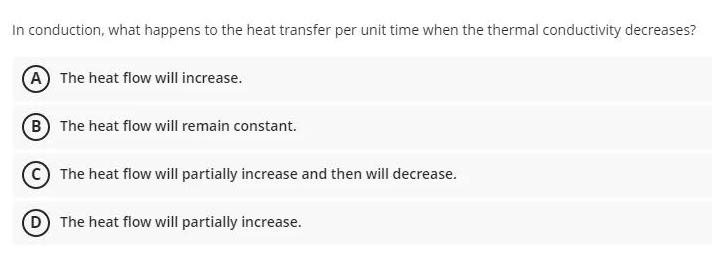Physics
Thermodynamics
In conduction, what happens to the heat transfer per unit time when the thermal conductivity decreases? (A) The heat flow will increase. (B) The heat flow will remain constant. (C)The heat flow will partially increase and then will decrease. (D) The heat flow will partially increase.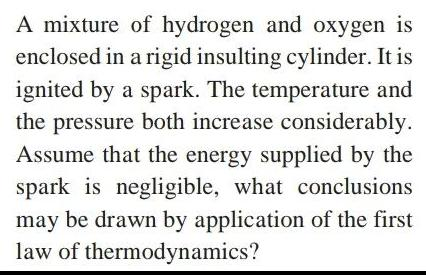Physics
Thermodynamics
A mixture of hydrogen and oxygen is enclosed in a rigid insulting cylinder. It is ignited by a spark. The temperature and the pressure both increase considerably. Assume that the energy supplied by the spark is negligible, what conclusions may be drawn by application of the first law of thermodynamics?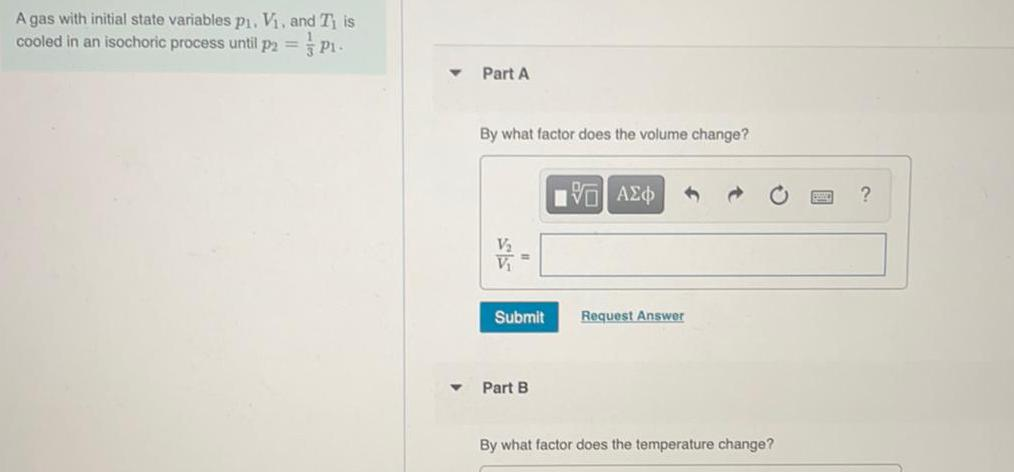Physics
Thermodynamics
A gas with initial state variables pi. V₁, and Ti is cooled in an isochoric process until p₂ =1/3 P₁. By what factor does the volume change? By what factor does the temperature change?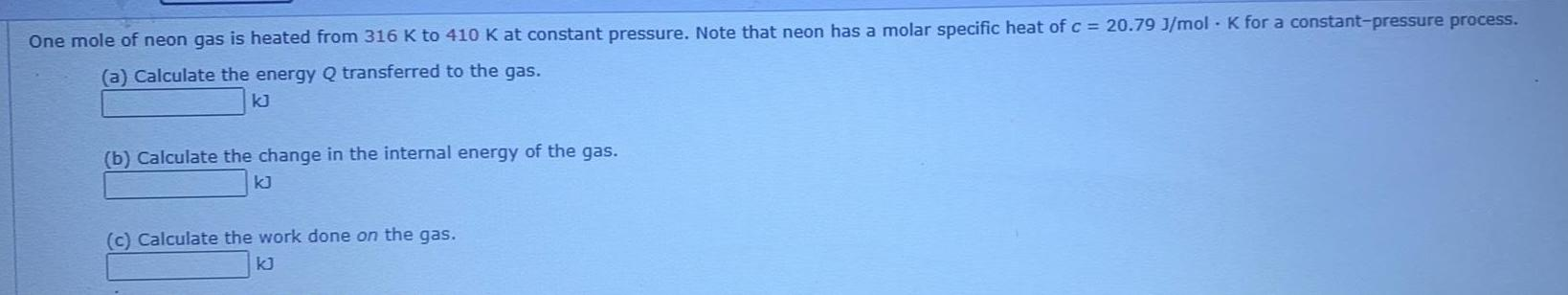Physics
Thermodynamics
One mole of neon gas is heated from 316 K to 410 K at constant pressure. Note that neon has a molar specific heat of c = 20.79 J/mol K for a constant-pressure process. (a) Calculate the energy Q transferred to the gas. (b) Calculate the change in the internal energy of the gas. (c) Calculate the work done on the gas.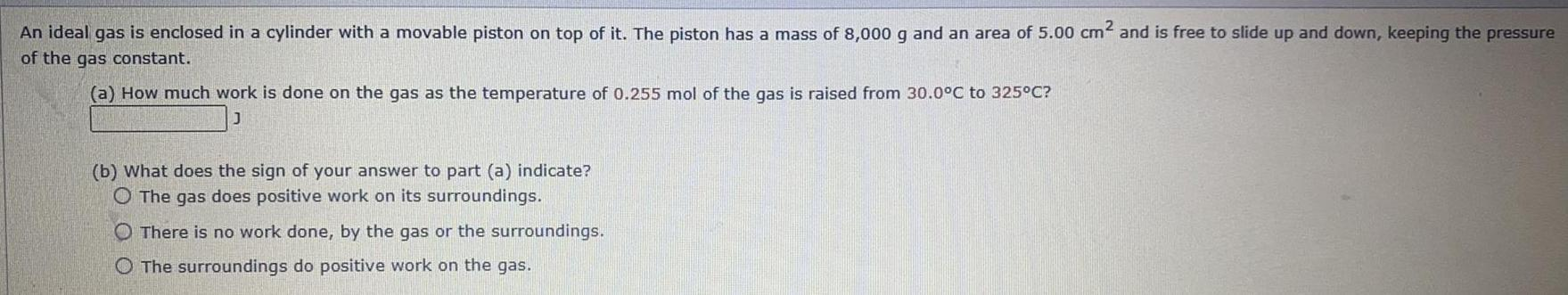Physics
Thermodynamics
An ideal gas is enclosed in a cylinder with a movable piston on top of it. The piston has a mass of 8,000 g and an area of 5.00 cm² and is free to slide up and down, keeping the pressure of the gas constant. (a) How much work is done on the gas as the temperature of 0.255 mol of the gas is raised from 30.0°C to 325°C? _____J (b) What does the sign of your answer to part (a) indicate? The gas does positive work on its surroundings. There is no work done, by the gas or the surroundings. The surroundings do positive work on the gas.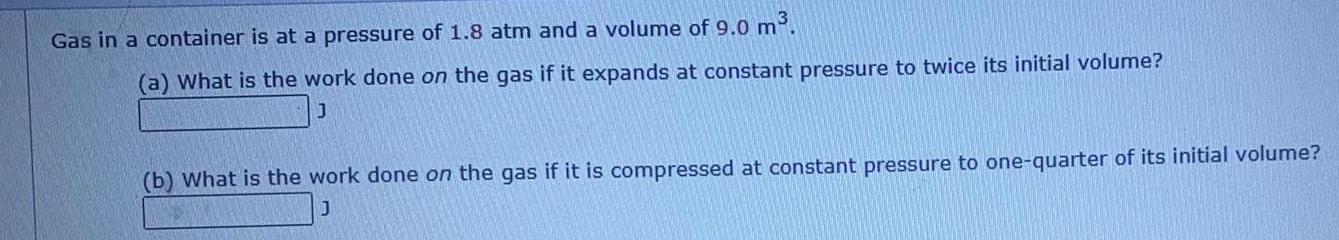Physics
Thermodynamics
Gas in a container is at a pressure of 1.8 atm and a volume of 9.0 m³. (a) What is the work done on the gas if it expands at constant pressure to twice its initial volume? (b) What is the work done on the gas if it is compressed at constant pressure to one quarter of its initial volume?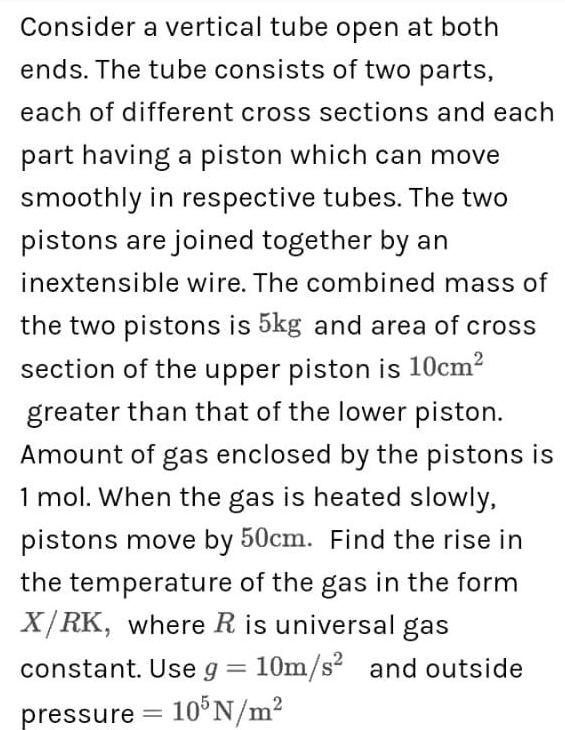Physics
Thermodynamics
Consider a vertical tube open at both ends. The tube consists of two parts, each of different cross sections and each part having a piston which can move smoothly in respective tubes. The two pistons are joined together by an inextensible wire. The combined mass of the two pistons is 5kg and area of cross section of the upper piston is 10cm² greater than that of the lower piston. Amount of gas enclosed by the pistons is 1 mol. When the gas is heated slowly, pistons move by 50cm. Find the rise in the temperature of the gas in the form X/RK, where R is universal gas constant. Use g = 10m/s² and outside pressure = 105 N/m²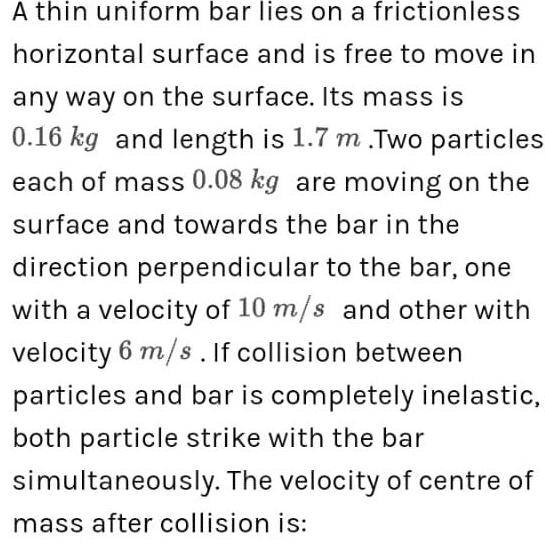Physics
Thermodynamics
A thin uniform bar lies on a frictionless horizontal surface and is free to move in any way on the surface. Its mass is 0.16 kg and length is 1.7 m .Two particles each of mass 0.08 kg are moving on the surface and towards the bar in the direction perpendicular to the bar, one with a velocity of 10 m/s and other with velocity 6 m/s. If collision between particles and bar is completely inelastic, both particle strike with the bar simultaneously. The velocity of centre of mass after collision is: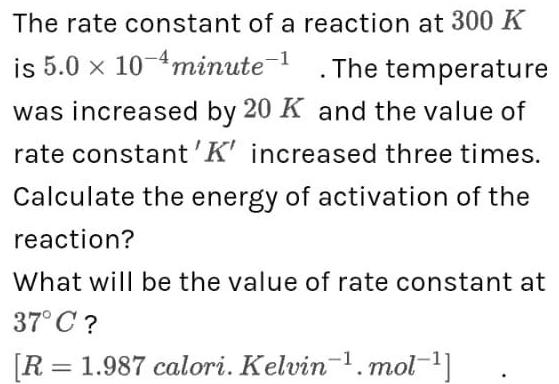Physics
Thermodynamics
The rate constant of a reaction at 300 K is 5.0 × 10-minute-¹ . The temperature was increased by 20 K and the value of rate constant'K' increased three times. Calculate the energy of activation of the reaction? What will be the value of rate constant at 37°C ?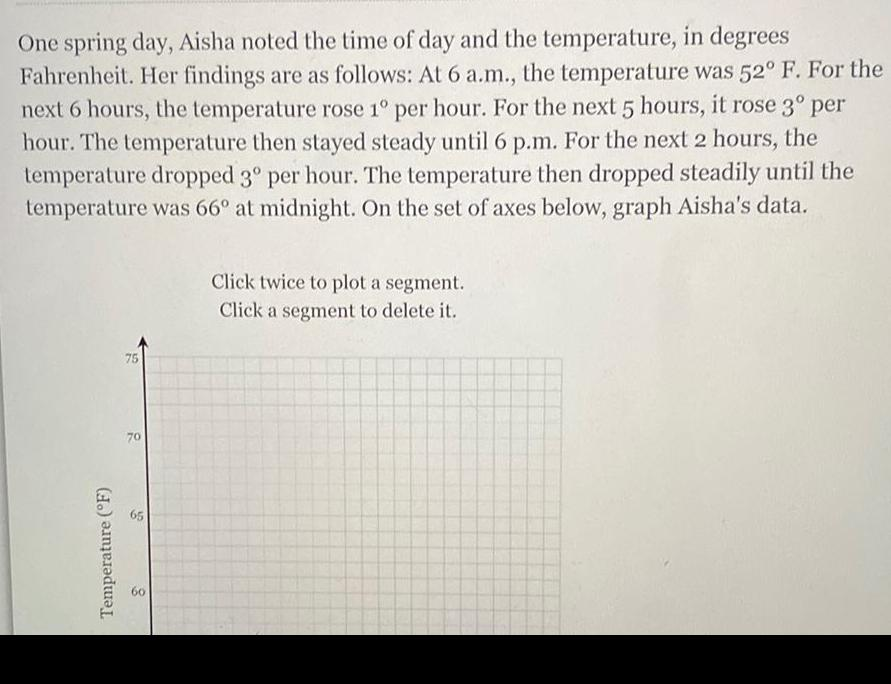Physics
Thermodynamics
One spring day, Aisha noted the time of day and the temperature, in degrees Fahrenheit. Her findings are as follows: At 6 a.m., the temperature was 52° F. For the next 6 hours, the temperature rose 1° per hour. For the next 5 hours, it rose 3° per hour. The temperature then stayed steady until 6 p.m. For the next 2 hours, the temperature dropped 3° per hour. The temperature then dropped steadily until the temperature was 66° at midnight. On the set of axes below, graph Aisha's data.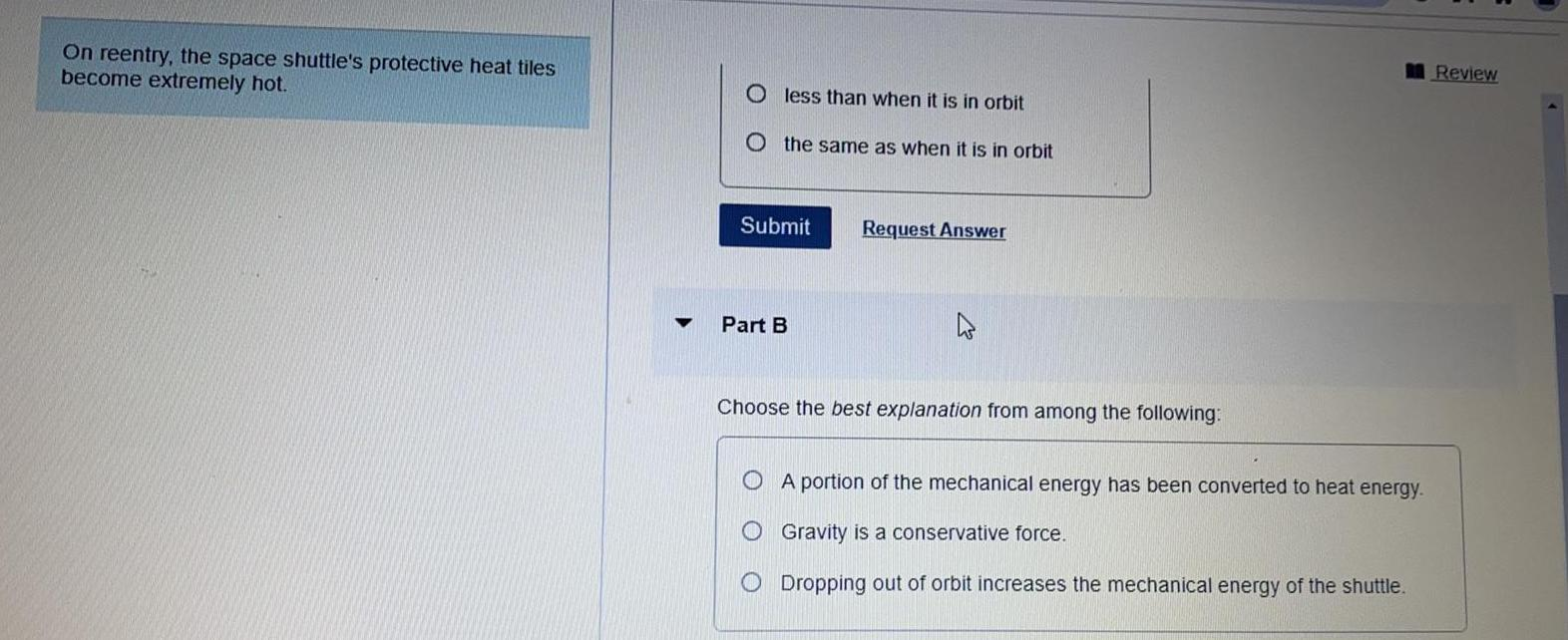Physics
Thermodynamics
On reentry, the space shuttle's protective heat tiles become extremely hot. less than when it is in orbit the same as when it is in orbit Choose the best explanation from among the following: A portion of the mechanical energy has been converted to heat energy. Gravity is a conservative force. Dropping out of orbit increases the mechanical energy of the shuttle. Review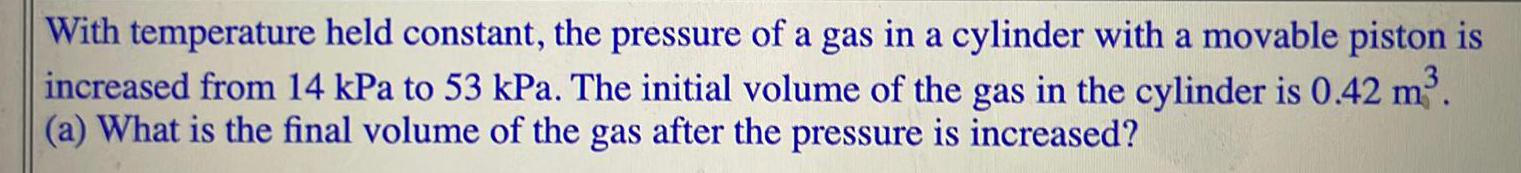Physics
Thermodynamics
With temperature held constant, the pressure of a gas in a cylinder with a movable piston is increased from 14 kPa to 53 kPa. The initial volume of the gas in the cylinder is 0.42 m³. (a) What is the final volume of the gas after the pressure is increased?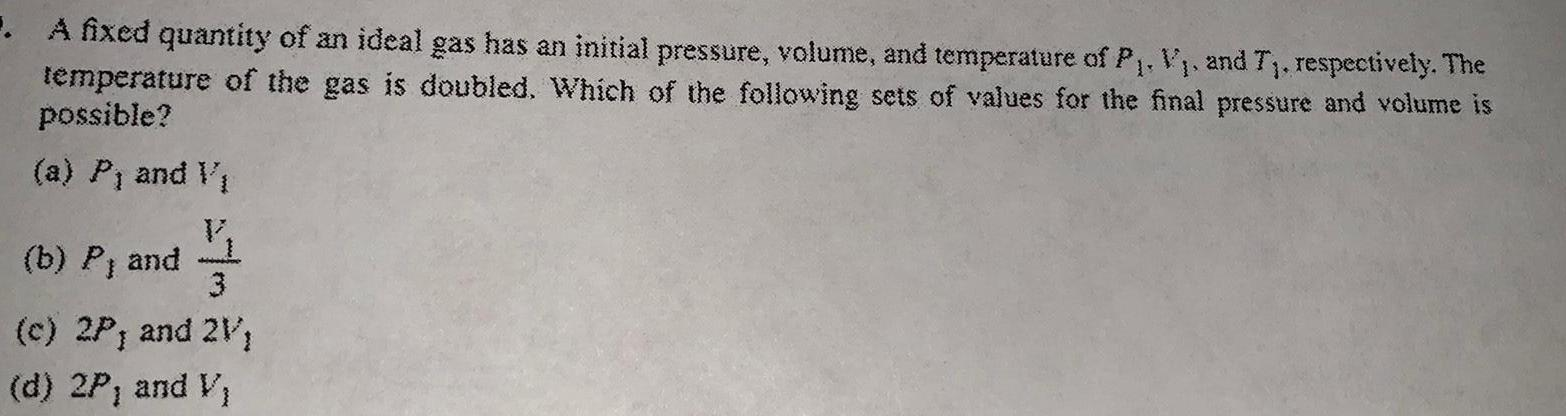Physics
Thermodynamics
. A fixed quantity of an ideal gas has an initial pressure, volume, and temperature of P₁, V₁, and T₁, respectively. The temperature of the gas is doubled. Which of the following sets of values for the final pressure and volume is possible? (a) P₁ and V₁ (b) P, and 3 (c) 2P, and 2V; (d) 2P, and V₁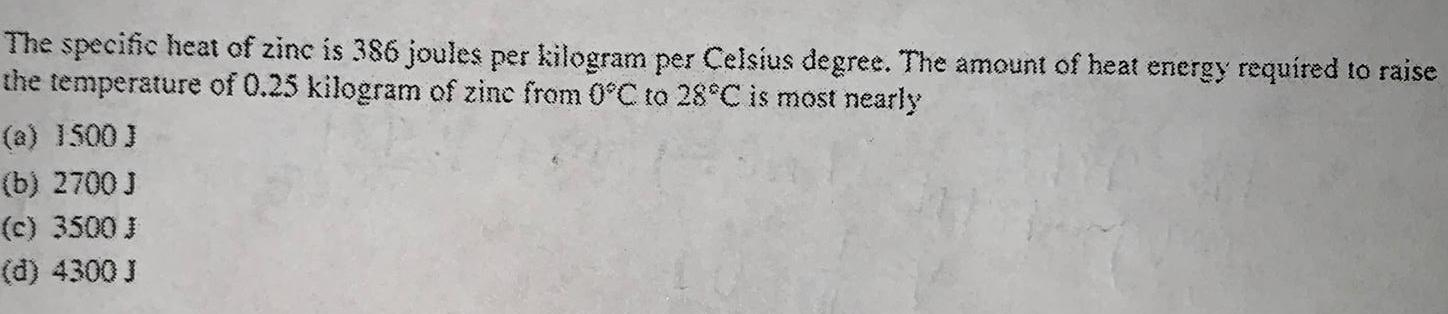Physics
Thermodynamics
The specific heat of zinc is 386 joules per kilogram per Celsius degree. The amount of heat energy required to raise the temperature of 0.25 kilogram of zinc from 0°C to 28°C is most nearly (a) 1500 J (b) 2700 J (c) 3500 J (d) 4300 J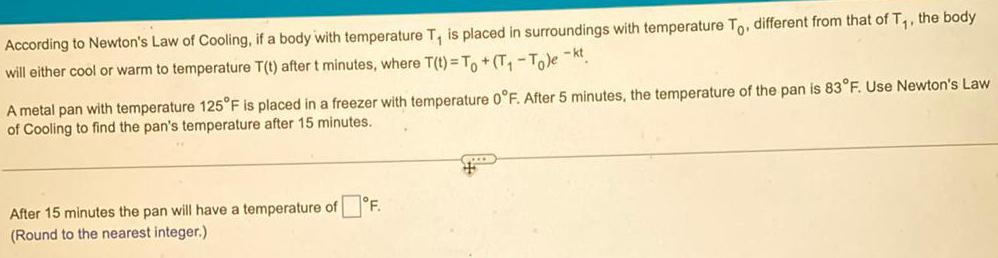Physics
Thermodynamics
According to Newton's Law of Cooling, if a body with temperature T₁ is placed in surroundings with temperature To. different from that of T₁, the body will either cool or warm to temperature T(t) after t minutes, where T(t)= To +(T₁-To)ekt. A metal pan with temperature 125°F is placed in a freezer with temperature 0°F. After 5 minutes, the temperature of the pan is 83°F. Use Newton's Law of Cooling to find the pan's temperature after 15 minutes. After 15 minutes the pan will have a temperature of (Round to the nearest integer.) F.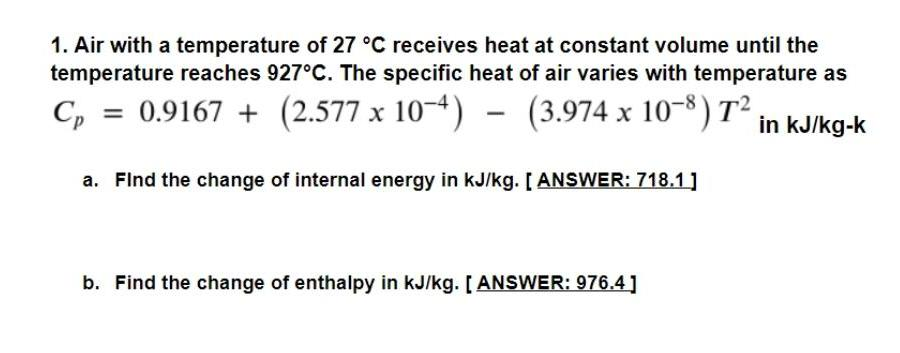Physics
Thermodynamics
Air with a temperature of 27 °C receives heat at constant volume until the temperature reaches 927°C. The specific heat of air varies with temperature as Cp = 0.9167 + (2.577 x 10^-4) (3.974 x 10^-8) T² in kJ/kg-k a. Find the change of internal energy in kJ/kg. b. Find the change of enthalpy in kJ/kg.